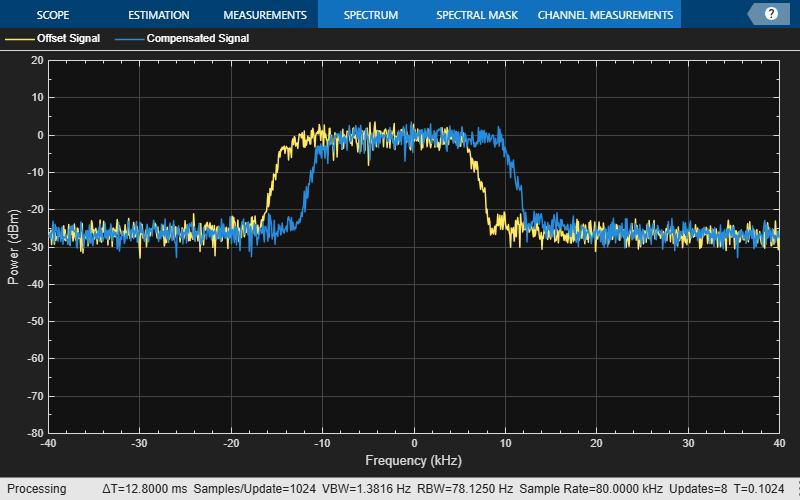# info

Characteristic information about coarse frequency compensator

## Syntax

``infostruct = info(coarseFreqComp)``

## Description

example

````infostruct = info(coarseFreqComp)` returns characteristic information for the specified coarse frequency compensator.```

## Examples

collapse all

Compensate for a 4 kHz frequency offset imposed on a noisy QPSK signal.

Set up the example parameters.

```nSym = 2048; % Number of input symbols sps = 4; % Samples per symbol nSamp = nSym*sps; % Number of samples fs = 80000; % Sampling frequency (Hz)```

Create a square root raised cosine transmit filter.

```txfilter = comm.RaisedCosineTransmitFilter( ... RolloffFactor=0.2, ... FilterSpanInSymbols=8, ... OutputSamplesPerSymbol=sps);```

Create a phase frequency offset object to introduce the 4 kHz frequency offset.

```freqOffset = comm.PhaseFrequencyOffset( ... FrequencyOffset=-4000, ... SampleRate=fs);```

Create a coarse frequency compensator object to compensate for the offset.

```freqComp = comm.CoarseFrequencyCompensator( ... Modulation="qpsk", ... SampleRate=fs, ... FrequencyResolution=1);```

Generate QPSK symbols, filter the modulated data, pass the signal through an AWGN channel, and apply the frequency offset.

```data = randi([0 3],nSym,1); modData = pskmod(data,4,pi/4); txSig = txfilter(modData); rxSig = awgn(txSig,20,"measured"); offsetData = freqOffset(rxSig);```

Compensate for the frequency offset using the coarse frequency compensator. When the frequency offset is high, applying coarse frequency compensation prior to receive filtering is benefitial because filtering suppresses energy in the useful spectrum.

`[compensatedData,estFreqOffset] = freqComp(offsetData);`

Display the estimate of the frequency offset.

`estFreqOffset`
```estFreqOffset = -4.0001e+03 ```

Return information about the coarse frequency compensator System object. To obtain the FFT length, you must call coarse frequency compensator System object prior to calling the `info` object function.

`freqCompInfo = info(freqComp)`
```freqCompInfo = struct with fields: FFTLength: 131072 Algorithm: 'FFT-based' ```

Create a spectrum analyzer object and plot the offset and compensated spectra. Verify that the compensated signal has a center frequency at 0 Hz and that the offset signal has a center frequency at -4 kHz.

```sa = spectrumAnalyzer( ... SampleRate=fs, ... ShowLegend=true, ... ChannelNames=["Offset Signal","Compensated Signal"]); sa([offsetData compensatedData]) release(sa)```## Input Arguments

collapse all

Coarse frequency compensator specified as a `comm.CoarseFrequencyCompensator` System object.

## Output Arguments

collapse all

Characteristic information about coarse frequency compensator, returned as a structure containing these fields.

Number of fast Fourier transform (FFT) samples, returned as a scalar. Appears only when `Algorithm` is `FFT-based`.

#### Dependency

To enable this field, set the `Algorithm` property of the `coarseFreqComp` input to `'FFT-based'`.

Algorithm used to estimate frequency offset, returned as `'FFT-based'` or `'Correlation-based'`. This value matches the `Algorithm` property of the `coarseFreqComp` input.

Number of samples used to estimate the autocorrelation, returned as a positive integer.

#### Dependency

To enable this field, set the `Algorithm` property of the `coarseFreqComp` input to `'Correlation-based'`.

Data Types: `struct`

## Version History

Introduced in R2015b